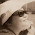## Wednesday, 30 January 2013

### 20130130 Lesson Activity (1) Review - Ordering of Numbers

In the last lesson, each group was given a stack of cards (12 pieces) to arrange them in ascending order.

2 groups submitted. The order of the cards presented by Group 3 & Group 4 are almost identical except the last 2 cards.

Before revealing the answer... We need to know the strategy when dealing with such questions:
Key words: ascending order
Strategy:
Convert all numbers to the "same format" for comparison; make reference to a number line.

Point to Ponder: Do you know how to obtain the value of π from the calculator?

Hence, the answer by Group 3 is correct.
(click at link to view Group 3's answer)

1.Just press the 'pi' sign in the calculator

1.2.I believe the 'Pi' sign is π.You just press Alt+P

2.Theres a pie sign on the caculator

1.3.press the 'pi' button on the calculator.

4.For calculator model fx-95SG PLUS,press 'shift' and the button 'X10' which is just beside the ''=''button.

5.press the pi button on the calculator and then compare the third decimal place of the pi and (22 divided by 7) as the numbers in the first two decimal places are the same and they cannot be compared.

6.you can either use 22/7 or click on the pi button of the calculator

7.You have to press the pi sign on the calculator or 22/7 depending on the question.

8.Press the PI sign on the calculator, or use 3.141 or 22/7

9.Just press the pi sign on the calculator, use 3.147... or use 22/7

10.Depending on what the qn requires

11.just press the pi sign on the calculator.

12.There's a pi sign on the calculator

13.I obtain PI by pressing SHIFT, followed by X10x. (For my calculator)

14.There is a Pi sign on the calclator